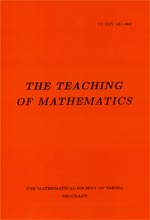﻿ The Teaching of Mathematics - Instructions for Authors ﻿
 THE TEACHING OF MATHEMATICSGeometrical definition of $\pi$ and its approximations by nested radicals Milan Janjić AbstractIn this paper the length of the arc of a circle and the area of a circular sector are defined in an elementary way. From this we derive the geometrical definition of the number $\pi$, in two equivalent ways. Formulas for the area and the perimeter of a circle are proved. Also, the number $\pi$ is represented as a limit of several sequences involving nested radicals. From this some approximations of $\pi$ are obtained. One of them is in terms of Golden ratio.Keywords: Circle; circular sector; area; length. Pages:  25$-$34 Volume  XI ,  Issue  1 ,  2008

﻿